# RBSE Maths Class 11 Chapter 13: Important Questions and Solutions

RBSE Maths Chapter 13 – Limits and derivatives Class 11 Important questions and solutions are available here. The important questions and solutions of Chapter 13, available at BYJU’S, contain step by step explanations along with the solutions. All these important questions are based on the new pattern prescribed by the RBSE. Students can also get the syllabus and textbooks on RBSE Class 11 solutions.

Chapter 13 of the RBSE Class 11 Maths will help the students to solve problems related to the definition of a limit of a function, the existence of a limit, theorems on limits, method of evaluation of limits, derivatives, algebra of derivatives of functions. After referring to these important questions and solutions, you can refer to 2020-2021 solutions for the chapter here.

### RBSE Maths Chapter 13: Exercise 13.1 Textbook Important Questions and Solutions

Question 1: Show that left and right limits of function f (x) = [logex] / [x – 1] at x = 1 are equal and their value is 1.

Solution: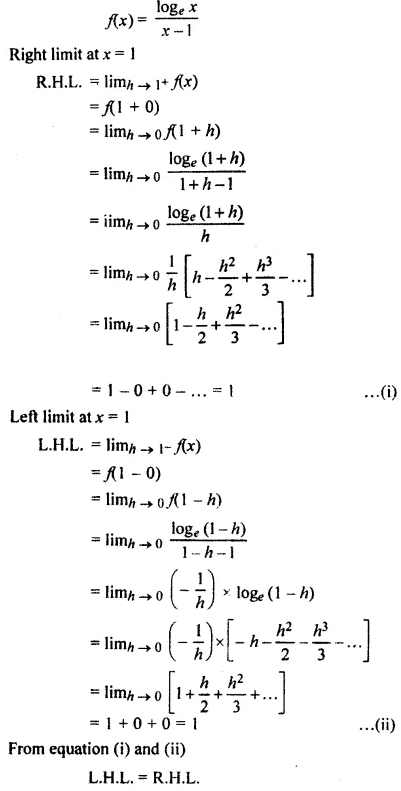Hence, the left and right limits of the given function at x = 1 are equal and their value is 1.

Question 2: Is limit of the function f (x) = [x + |x|] / [x] at x = 0?

Solution: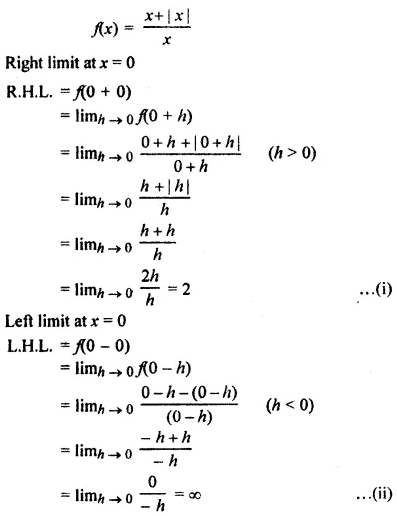From equations (i) and (ii),

L.H.L. ≠ R.H.L.

⇒ f (0 – 0) ≠ x (0 + 0)

Hence, the limit of function does not exist at x = 0.

Question 3: Prove that at x = 0, limits of function f (x) = | x | + | x – 1| exists.

Solution:

f(x) = | x | + | x – 1 |

Right limit at x = 0

R.H.L. = limx→0+ + f (x)

= f (0 + 0)

= limh→0 f (0 + h)

= limh→0 |0 + h| + |0 + h – 1 | (h > 0)

= limh→0 | h | + | h – 1 |

= 0 + | 0 – 1 | = 1 …(i)

Left limit at x = 0

L.H.L. = limh→0– f (x) = f (0 – 0)

= limh→0 f (0 – h)

= limh→0 | 0 – h | + | 0 – h – 1 |

= limh→0 | 0 – h | + | – (h – 1) |

= | -0 | + | – (0 + 1) |

= 0 + 1

= 1 …(ii)

It is clear from equation (i) and (ii),

L.H.L. = R.H.L.

⇒ f (0 – 0)

= f (0 + 0)

= 1

Hence, the limit of the function exists at x = 0.

Hence proved.

Question 4: Prove that at x = 2, limits of function do not exist.

$$\begin{array}{l}f(x)=\left\{\begin{matrix} x^{2}+x+1 &if \ x\geq 2 \\ x& if \ x<2 \end{matrix}\right.\end{array}$$

Solution:

$$\begin{array}{l}f(x)=\left\{\begin{matrix} x^{2}+x+1 &if \ x\geq 2 \\ x& if \ x<2 \end{matrix}\right.\end{array}$$

Right limit at x = 2

R.H.L. = limx→0+ f (x)

= f (2 + 0) = limh→0 f (2 + h)

= limh→0 [(2 + h)2 + (2 + h) + 1]

= limh→0 [4 + h2 + 4h + 2 + h + 1]

= limh→0 [h2 + 5h + 7]

= 02 + 5 (0) + 7

= 7 ….(i)

Left limit at x = 2

L.H.L. = limx→0– f (x) = f (2 – 0)

= limh→0 f (2 – h)

= limh→0 [2 – h]

= 2 – 0

= 2

From equations (i) and (ii),

L.H.L. ≠ R.H.L.

⇒ f (2 – 0) ≠ f (2 + 0)

Hence, the limit of function does not exist at x = 2.

Question 5: Find the left and right limit of function f (x) = x cos (1 / x) at x = 0.

Solution: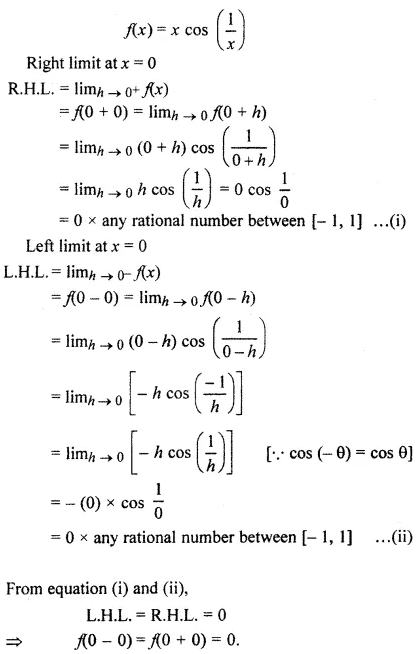### RBSE Maths Chapter 13: Exercise 13.2 Textbook Important Questions and Solutions

Question 1: Evaluate the following limits.

[i] limx→2 [x2 – 3x + 2] / [x2 + x – 6]

[ii] limx→1 [(2x + 3) (x – 1)] / [2x2 + x – 3]

Solution: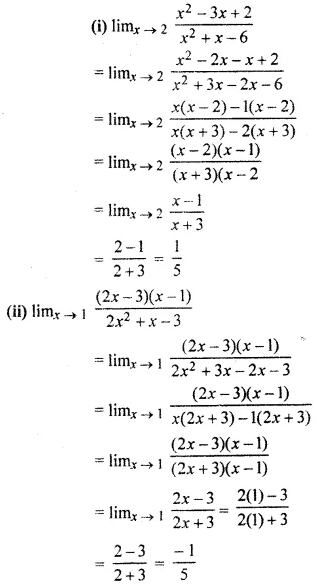Question 2: Evaluate the limits.

[i] limɑ→π/4 [sin ɑ – cos ɑ] / [ɑ – π/4]

[ii] limx→1 [xn – 1] / [x – 1]

Solution: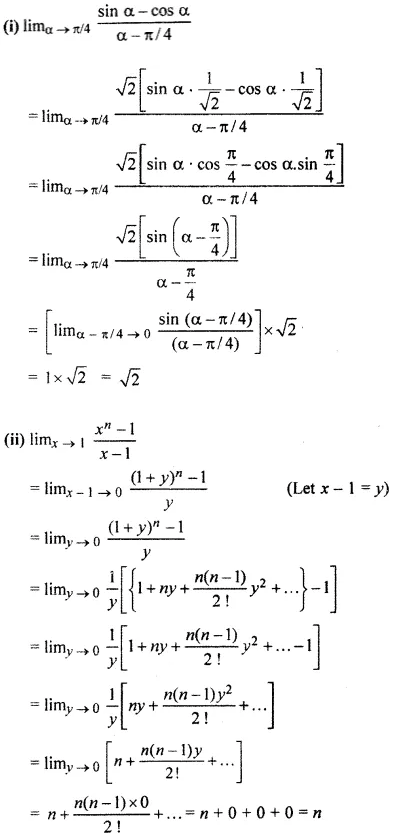Question 3:

[i] limx→0 [2x – 1] / √(1 + x) – 1

[ii] limx→0 {[√1 + x2] – [√1 – x2]} / {x2}

Solution: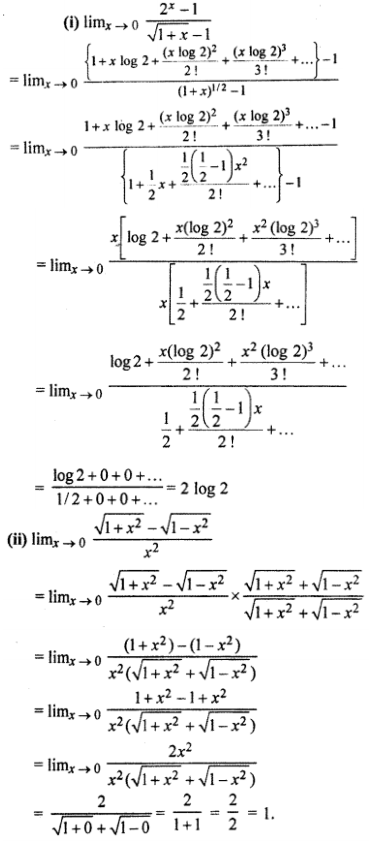Question 4:

[i] limx→0 {ex + e-x – 2 cosx} / {x sinx}

[ii] limx→0 {sin-1 x – tan-1 x} / {x3}

Solution: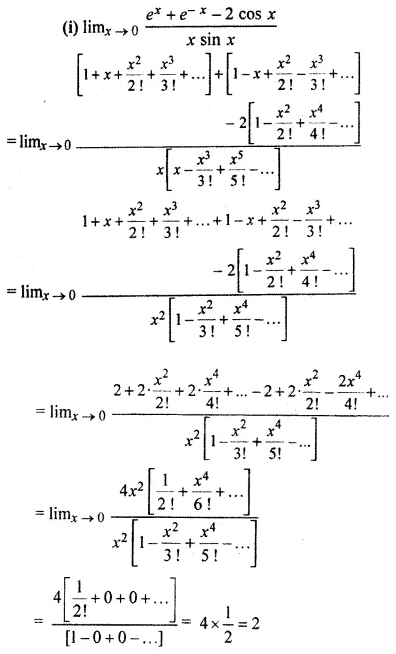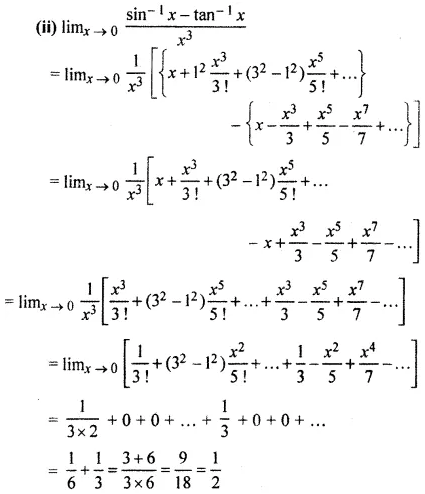Question 5:

[i] limx→∞ [√{x + sinx} / {x – cosx}]

[ii] limn→∞ [√(3n2 – 1) – √(2n2 – 1)] / [4n + 3]

Solution: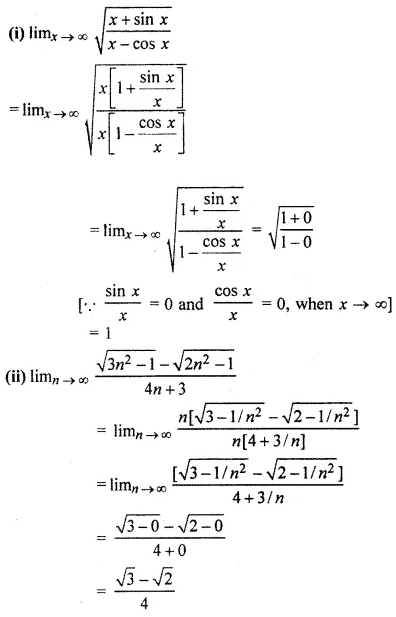Question 6:

[i] limx→0 [sin 5x – sin 3x] / [sin x]

[ii] limx→0 [esinx – 1] / [x]

Solution: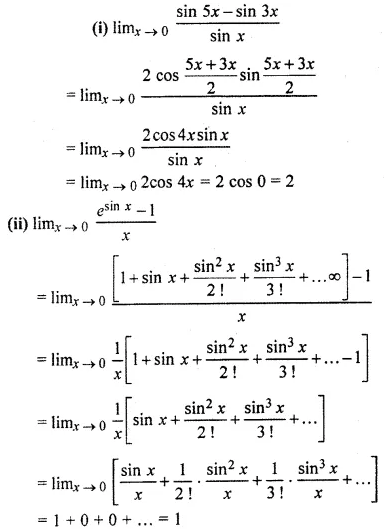Question 7:

[i] limx→0 [x (ex – 1)] / [1 – cos x]

[ii] limx→a {[x + 2]3/2 – [a + 2]3/2} / [x – a]

Solution: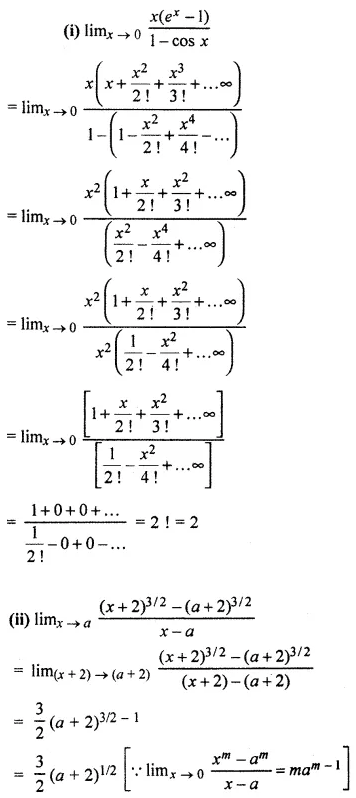### RBSE Maths Chapter 13: Exercise 13.3 Textbook Important Questions and Solutions

Question 1: Find the derivative of 49x at x = 50.

Solution: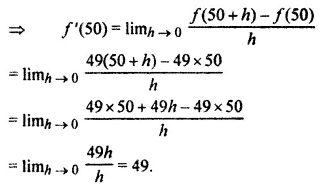Question 2: Find the derivative of the function x3 – 16 from the first principle.

Solution:

Let y = x3 – 16

Again, let y + δy = (x + δx)3 – 16

⇒ δy = (x + δx)3 – 16 – y

⇒ δy = (x + δx)3 – 16 – x3 + 16

⇒ δy = (x + δx)3 – x3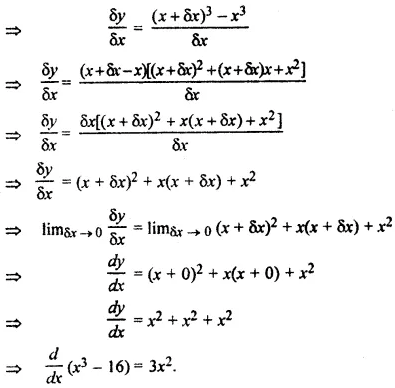### RBSE Maths Chapter 13: Miscellaneous Questions and Solutions

Question 1: Find the value of limx→1 [x – 1] / [2x2 – 7x + 5].

Solution: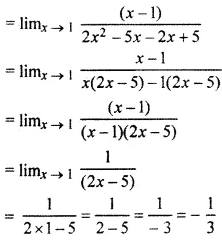Question 2: Find the value of limx→∞ [sinx] / [x].

Solution: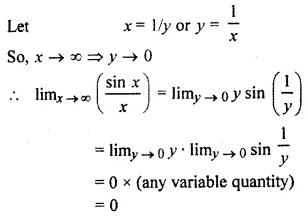Question 3: Find the value of limx→0 [xex – loge[1 + x]] / x2.

Solution: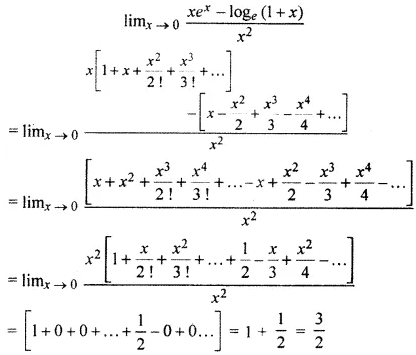Question 4: Find the value of limn→∞ [(1 / 2) + (1 / 22) + (1 / 23) + …… (1 / 2n)].

Solution: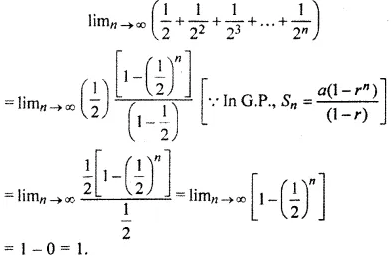Question 5: Find the value of limx→∞ [x sin (𝛑 / 4x) cos (𝛑 / 4x)].

Solution: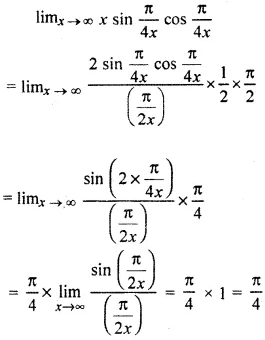Question 6: Find the value of limx→0 [ax – bx] / [x].

Solution: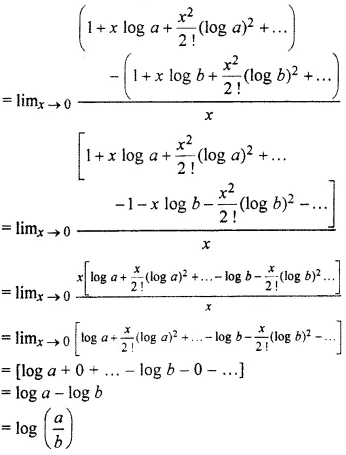Question 7: The value of limx→0 [sin x0 / x] is

Solution: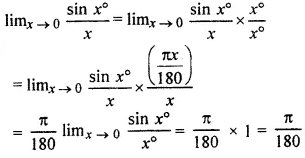Question 8: The value of limx→0 [1 – cosx] / [x2] is

Solution: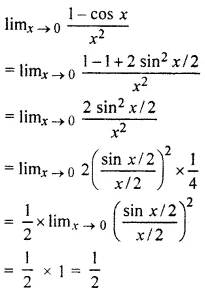Question 9: The value of limx→0 [sin 3x / tan x]4 is

Solution: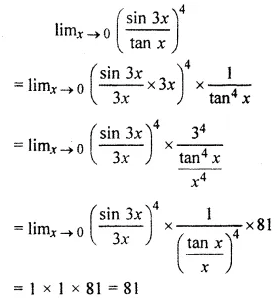Question 10: The value of limx→0+ [1 + 31/x] / [1 – 31/x] is

Solution: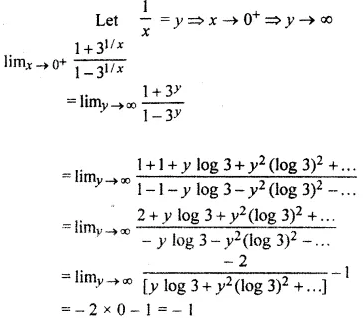Question 11: The derivative of xn is

Solution:

Let y = xn

d / dx (y) = d / dx (xn) = nxn-1

d / dx (xn) = nxn-1

Question 12: The derivative of 1 / √x is

Solution:

Let y = 1 / √x or 1 / x1/2 or x

dy / dx = d / dx (x)

= [-1 / 2] x-½-1

= [-1 / 2] x-3/2

= [-1 / 2x3/2]

= -[1 / 2x √x]

Question 13: The derivative of 5x is

Solution:

(5x) = 5x loge5

Question 14: The derivative of (loga x) is

Solution:

d / dx (loga x)

= d / dx (log x / log a)

= [1 / log a] * [d / dx] (log x)

= (1 / x) log a

Question 15: If f (x) = x3 + 6x2 – 5 then f'(1) is equal to

Solution:

f (x) = x3 + 6x2 – 5

⇒ f’ (x) = 3x2 + 12x – 0

⇒ f’ (x) = 3x2 + 12x

⇒ f’ (1) = 3(1)2 + 12(1)

⇒ f’ (1) = 3 + 12

= 15

Question 16: Find the derivative of [sec x0].

Solution:

Let y = sec x0

dy / dx = sec x0 tan x0 (d / dx) (x0)

dy / dx = sec x0 tan x0 (d / dx) (𝛑x / 180o)

dy / dx = sec x0 tan x0 (d / dx) (𝛑 / 180o)

Question 17: Find the derivative of [logx a].

Solution: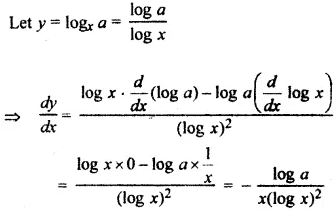Question 18: If f (x) = [2x + c] / [x – 1] and f’ (0) = 0, then find the value of c.

Solution: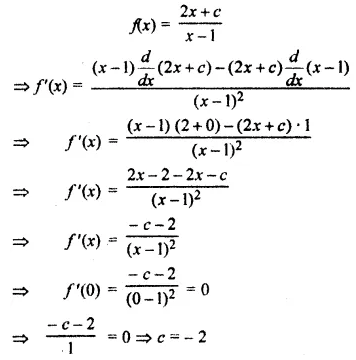Question 19: The derivative of loge √x is

Solution:

Let y = loge √x

dy / dx = d / dx (loge √x)

= [1 / √x] * (d / dx) (√x)

= [1 / √x] * (d / dx) (x½)

= [1 / √x] * (1 / 2) x1/2-1

= [1 / √x] * (1 / 2) x-1/2

= [1 / √x] * (1 / 2) * (1 / √x)

= 1 / 2x

Question 20: Find the values of a,b and c in limx→0 [aex – b cos x + ce-x] / [x sin x] = 2.

Solution: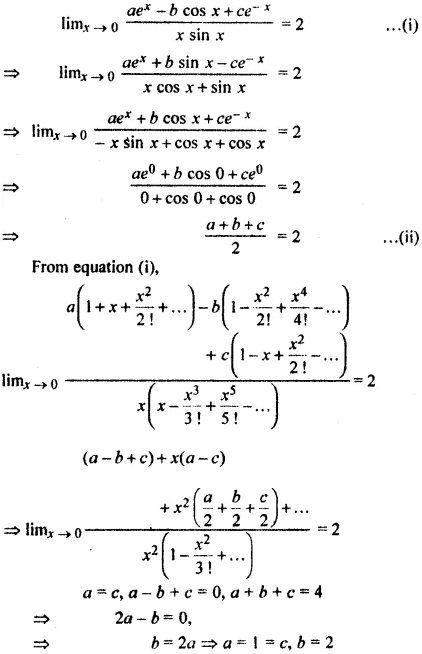Question 21: limx→∞ √x (√x + c – √x) =

Solution: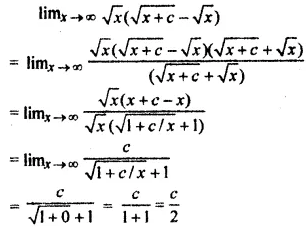Question 22: limx→1 {[√x – 1] + [√x – 1]} / [√x2 – 1]

Solution: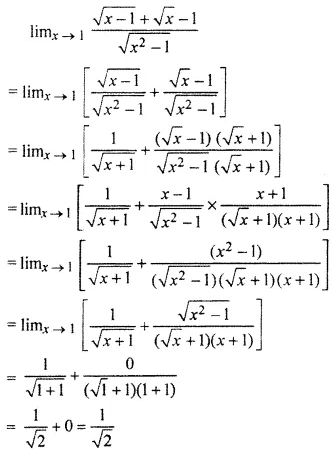Question 23: Evaluate the limit limx→∞ {[3x2 + 2x +1] / [x2 + x + 2]}[6x+1]/[3x-1]

Solution: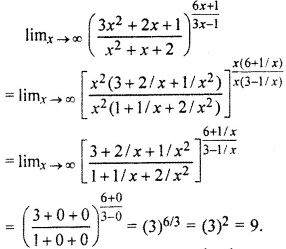Question 24: limx→∞ 2x sin [a / 2x]

Solution: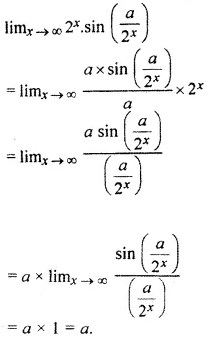Question 25: limx→1 [ex – e-x] / [ex + e-x]

Solution: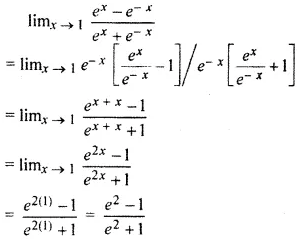Question 26: limx→𝛑/2 [1 + cos 2x] / [𝛑 – 2x)2

Solution: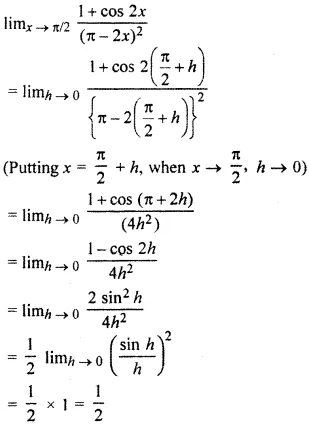Question 27: If y = [x] / [x + 5], then prove that x (dy / dx) = y (1 – y).

Solution:

y = [x] / [x + 5] —- (1)

xy + 5y = x

On differentiating,

x * (dy / dx) + y * 1 + 5 * (dy / dx) = 1

(x + 5) (dy / dx) + y = 1

(dy / dx) = (1 – y) / (x + 5)

Multiplying both sides by x,

x * (dy / dx) = [x] / [x + 5] * (1 – y)

x * (dy / dx) = y (1 – y) [from equation (1)]

Question 28: If y = x3 * ex (sin x), then find (dy / dx).

Solution:

y = x3 * ex (sin x)

On differentiating,

dy / dx = x3 * (d / dx) (ex sinx) + (ex sinx) * (d/ dx) (x3)

dy / dx = x3 * I + ex sinx * (3x2) [Let I = (d / dx) (ex sinx)]

dy / dx = x3 * I + 3x2 * (ex sinx) —- (1)

I = (d / dx) (ex sinx)

I = ex (d / dx) sinx + sin x (d / dx) (ex)

I = ex * cosx + sinx * ex —- (2)

From equations (1) and (2)

dy / dx = x3 ex cosx + x3 ex sinx + 3x2 ex sinx